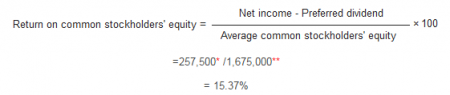# How to Calculate Return on Common Stockholders Equity Ratio?

Return on common stockholders equity ratio measures the success of a company in generating income for the benefit of common stockholders. It is computed by dividing the net income available for common stockholders by common stockholders’ equity. The ratio is usually expressed in percentage.

Formula:The numerator in the above formula consists of net income available for common stockholders which is equal to net income less dividend on preferred stock.

The denominator consists of average common stockholders’ equity which is equal to average total stockholders’ equity less average preferred stockholders equity.

If preferred stock is not present, the net income is simply divided by the average common stockholders’ equity to compute the common stock equity ratio.

Note for students: It is a better practice to use the average figures of common and preferred stock but if only closing figures are available, they can be used to compute common stockholders’ equity (denominator of the formula).

Example:Solution:*Net income available for common stockholders:
=\$329,500 – \$72,000
=\$257,500

**Average common stockholders’ equity:
=[(\$2,550,000 +\$2,400,000) / 2] – [(\$800,000 + \$800,000) / 2]
=\$2,475,000 – \$800,000
=\$1,675,000

Significance and Interpretation:

Return on common stockholders equity ratio shows how many dollars of net income have been earned for each dollar invested by the common stockholders. This ratio is a useful tool to measure the profitability from the owners’ view point because the common stockholders are considered the real owners of the corporation.

Like return on equity (ROE) ratio, a higher common stock equity ratio indicates high profitability and strong financial position of the company and can covert potential investors into actual common stockholders.